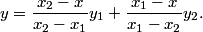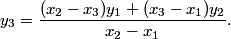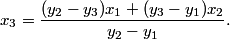# Linear interpolator help

## Troubleshooting interpolator

The interpolator is implemented in JavaScript. If you do not have JavaScript enabled in your browser, the form will not work.

The x input values must be unique and the y values must be unique. This means the same number cannot appear twice in the x column or twice in the y column.

Exactly one box must be empty before clicking the Calculate button.

## Interpolation derivations

A straight line through (x1, y1) and (x2, y2) satisfiesTo see why this is true, stick in x1 and x2 and see that you get y1 and y2 out. If a linear fit is correct at two different points, it's correct everywhere.

So if we set x equal to x3, thenSolving for x3 we see that## Understanding data

Trying to make sense of data? We can help.